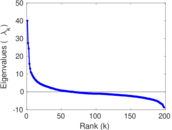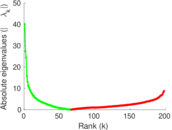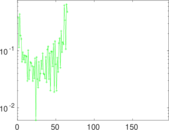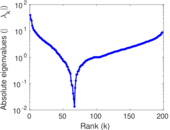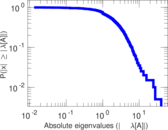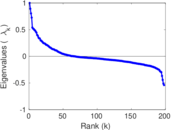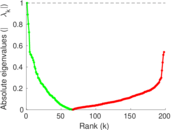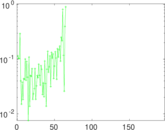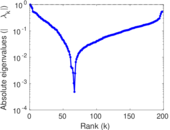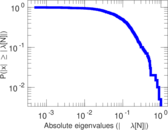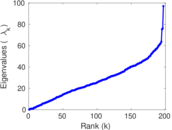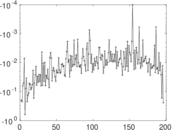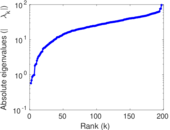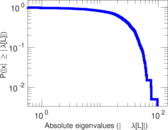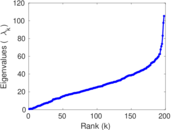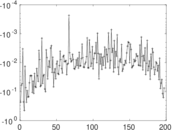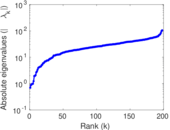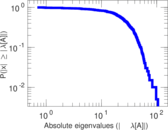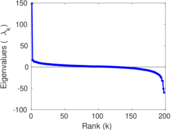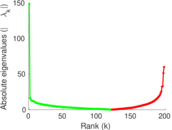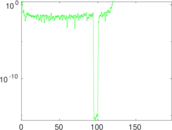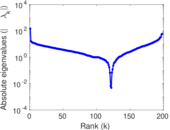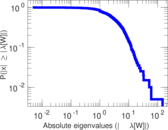# Jazz musicians

This is the collaboration network between Jazz musicians. Each node is a Jazz musician and an edge denotes that two musicians have played together in a band. The data was collected in 2003.

 Code `JZ` Internal name `arenas-jazz` Name Jazz musicians Data source http://deim.urv.cat/~alexandre.arenas/data/welcome.htm AvailabilityDataset is available for download Consistency checkDataset passed all tests Category Human social network Dataset timestamp 2003 Node meaning Musician Edge meaning Collaboration Network format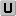Unipartite, undirected Edge typeUnweighted, no multiple edges LoopsDoes not contain loops Snapshot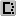Is a snapshot and likely to not contain all data Join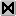Is the join of an underlying network

## Statistics

 Size n = 198 Volume m = 2,742 Loop count l = 0 Wedge count s = 103,212 Claw count z = 1,583,352 Cross count x = 21,666,963 Triangle count t = 17,899 Square count q = 406,441 4-Tour count T4 = 3,669,860 Maximum degree dmax = 100 Average degree d = 27.697 0 Fill p = 0.140 594 Size of LCC N = 198 Diameter δ = 6 50-Percentile effective diameter δ0.5 = 1.647 00 90-Percentile effective diameter δ0.9 = 2.793 55 Median distance δM = 2 Mean distance δm = 2.206 04 Gini coefficient G = 0.345 989 Balanced inequality ratio P = 0.373 450 Relative edge distribution entropy Her = 0.961 549 Power law exponent γ = 1.329 28 Tail power law exponent γt = 5.271 00 Tail power law exponent with p γ3 = 5.271 00 p-value p = 0.623 000 Degree assortativity ρ = +0.020 237 4 Degree assortativity p-value pρ = 0.134 010 Clustering coefficient c = 0.520 259 Spectral norm α = 40.027 4 Algebraic connectivity a = 0.571 994 Spectral separation |λ1[A] / λ2[A]| = 1.461 22 Non-bipartivity bA = 0.782 583 Normalized non-bipartivity bN = 0.460 437 Algebraic non-bipartivity χ = 0.692 773 Spectral bipartite frustration bK = 0.006 253 14 Controllability C = 1 Relative controllability Cr = 0.005 050 51

## Plots

### Fruchterman–Reingold graph drawing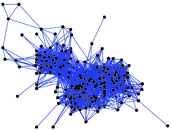### Degree distribution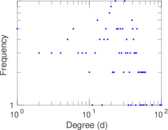### Cumulative degree distribution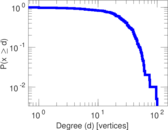### Lorenz curve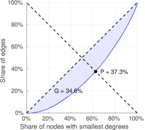### Spectral distribution of the adjacency matrix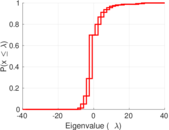### Spectral distribution of the normalized adjacency matrix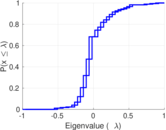### Spectral distribution of the Laplacian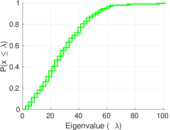### Spectral graph drawing based on the adjacency matrix### Spectral graph drawing based on the Laplacian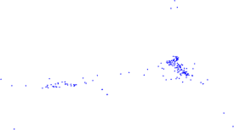### Spectral graph drawing based on the normalized adjacency matrix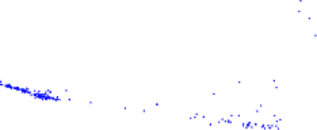### Degree assortativity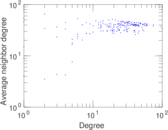### Zipf plot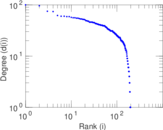### Hop distribution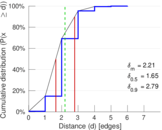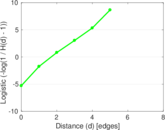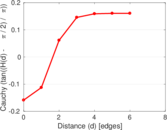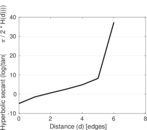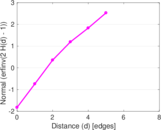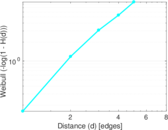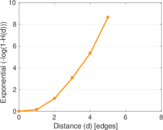### Double Laplacian graph drawing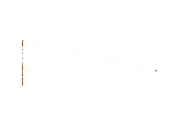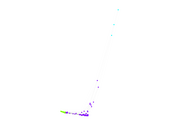### Delaunay graph drawing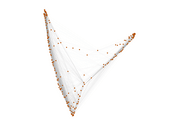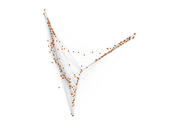### Clustering coefficient distribution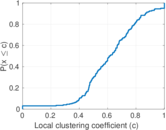### Average neighbor degree distribution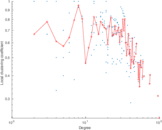### SynGraphy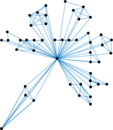### Matrix decompositions plots